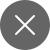# Linear Regression in Machine Learning- Detailed

5+

The beginners of Machine Learning(ML) start with the Linear Regression algorithm, personally speaking, while on my journey to learn Machine Learning, the first algorithm I encountered was this one.

The Linear Regression algorithm is a simple algorithm but has its good uses in the business segment. It can be used to predict the sales of a store or a product, it can be used to predict the price of houses, and so on.

Linear Regression is used basically to find the relationship between two or more continuous variables, it is of two types, let us all have a look:

1. Simple Linear Regression
2. Multiple Linear Regression

Linear regression establishes the relationship between two or more variables with the foundation of a straight line or the equation of a straight line which is

y= mx + c

Let us talk about Simple Linear Regression first:

### Simple Linear Regression

Finding a relationship between two continuous variables, where one variable is a predictor or an independent variable while the other one is a dependent variable or response variable. For eg to predict the net sales in dollars, one needs to know the total number of sales of a product, to predict the future production of the honey bee worldwide, one can predict it possibly if they know the amount of honey bee produced in the past years.

### Multiple Linear Regression

In business use cases multiple linear regression is mostly used because use cases are dependent on multiple variables that need to cater during the training of the linear regression model.

y= m1x1 + m2x2 + m3x3 + c

In both cases, y is the predictor while m & c are chosen so, they reduce the chance of error occurrence.

In mathematical terms, y=mx + c is a straight line, where, m is the slope of the line and c is the y-intercept. Intercept is that particular point where the graph meets the y-axis.

In regression terms, y is the predictor with m being the gradient. A gradient can either be positive or negative. Lines with a positive gradient are sloped in the upwards and the lines with a negative gradient are sloped in the downwards direction.

### Correlation of data

We have discussed above that linear regression establishes the relationship between two variables by drawing a line of best fit. The best way to understand the relationship between two variables is to find the correlation of data. The maximum value of the correlation of two variables is +1 and the minimum value is -1. A positive correlation value denotes a strong positive correlation between two variables, while a negative correlation between two variables denotes a negative correlation.

In the scatter plot below we can see a positive correlation between the two variables and hence we will draw out multiple lines, but only one line will be the line of best fit.

How are we going to find out the line of best fit?

The best fit line is the line that reduces the sum of error.

There are two methods to find the line of best fit:

1. Principle of Least Squares

### Principle of Least Squares

The line that satisfies the least square condition error is called the line of regression of y on x. To estimate y and x in a straight line y= mx+c, we need to find covariance between x & y.

Let the equation of straight line be:

y=mx+c

This straight line will try to approximate or define the linear relationship between x and y for the given dataset, by changing the values of m & c we can find the line of best fit.

What gradient descent does is define the cost function for parameters m & c and uses a suitable approach and make the best use to minimize the cost function. The cost function is a function that will minimize the parameters over a dataset. The most common function used in minimization of error is the mean squared error function.

### Performance evaluation of Linear Regression

Machine Learning models are trained and later on to there is a performance evaluation for every model so as to measure the performance of every model. In classification models like Logistic Regression, Naive Bayes, etc model performances like precision, recall, confusion matrix, etc are used(to learn confusion matrix click here). However, in Linear Regression models, the following are the 3 performance evaluation metrics:

1. R square measure
2. Mean Absolute Measure
3. Root Mean Square Error

The following three evaluation metrics shall be discussed in another article until then keep visiting this space for more tutorials related to Machine Learning.

### Pros & Cons of Linear Regression

#### Pros

Linear Regression models are easy to implement and learn as I have said at the beginning of the article as well, it is good for beginners to begin their journey in Machine Learning.

#### Cons

• The business use cases of Linear Regression is very limited, it cannot be implemented in a wide variety of business applications.
• The model is susceptible to outliers.5+

### 3 Responses

1. October 22, 2020

[…] you all understood what is Linear Regression and how to implement the same in […]

0
2. November 23, 2020

[…] ‘sales’ of a particular company based on its certain features, then many algorithms like Linear Regression and Decision Tree Regressor can be used. But both of these algorithms will make different […]

0
3. January 5, 2021

[…] (least absolute shrinkage and selection operator) is similar to linear regression. However, it uses the approach of absolute shrinkage and selection to reduce the value of […]

0